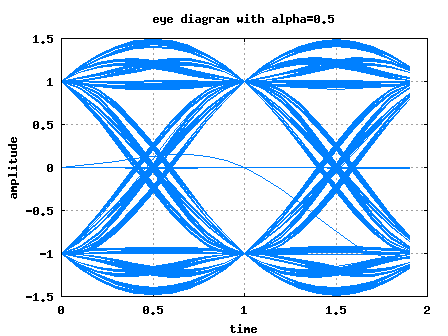- DSP log - http://www.dsplog.com -

Eye diagram with raised cosine filtering

Posted By Krishna Sankar On May 1, 2008 @ 8:03 am In Filter | 20 Comments

We have discussed about probable transmit pulse shaping filter and have observed that raised cosine filtering filtering  allows a simpler implementation, albeit at the cost of increased bandwidth. Let us know understand the eye diagram, which is a useful graphical tool to quantify the degradation of the signal due to filtering.

Eye diagram

An eye diagram is generated in an oscilloscope operating in the persistence mode by observing the output of the filter with the symbol timing serving as the trigger. The observation window can be set as 2 times the symbol period. (Refer. Section 5.1.3 in [DIG-COMM-BARRY-LEE-MESSERSCHMITT] ).

When the input data is random, the eye diagram which consists of many overlapped traces of the signal captures visually all the paths which the waveform takes.

Simulation script

Matlab/Octave script for simulating the eye diagram plot. The code performs the following

(a) Defines random BPSK modulated symbols (+1′s and -1′s)

(b) Defines two raised cosine filters with = 0.5, = 1

(c) Upsamples the transmit sequence by zero insertion

(d) Convolves the upsampled transmit sequence with the filter

(e) Overlays the time domain samples to plot the eye diagram

Update

25th May 2008

Corrected the issue. Modified the code to handle division by zero.

(a) for and

(b) for

(Thanks to the article in RFDesign.com, The care and feeding of digital, pulse-shaping filter , Ken Gentile)

19th May 2008

It has been brought to my attention that the code is unable to plot the eye diagram accurately in Matlab environment. The difference is because my version of Octave seems to handle the division by numbers close to zero cleanly, where as Matlab insists on returning Inf. I will fix the code and release an update. sorry for the inconvenience.Figure: Eye diagram following raised cosine filtering with = 0.5Figure: Eye diagram following raised cosine filtering with = 1

As can be observed from the above figures, the above waveform has a shape similar to the human eye and hence the name eye diagram.Observations

1. For increasing the margin for error free transmission, the vertical opening of the eye should be more. In the presence of inter-symbol interference, the vertical opening of the eye reduces, thus increasing the probability of error.

2. The ideal sampling instant is the point where the vertical eye opening is maximum.

3. Smaller horizontal eye opening means implies more sensitivity to timing errors.

Note:

From the above figures, it can be observed that the horizontal eye opening with =0.5 is smaller than with =1.

Reason: The tails of the raised cosine filter with =1 dies away faster than the case where =0.5. Hence error in timing cause a bigger performance degradation for =0.5 than for=1 scenario. However, the flip side of using =1 is the increased bandwidth required for transmission.

Reference

[DIG-COMM-BARRY-LEE-MESSERSCHMITT]Digital Communication: Third Edition, by John R. Barry, Edward A. Lee, David G. Messerschmitt 

Hope this helps.
KrishnaURL to article: http://www.dsplog.com/2008/05/01/eye-diagram-plot-matlab-raised-cosine-filter/

URLs in this post:

 raised cosine filtering filtering: http://www.dsplog.com/2008/04/22/raised-cosine-filter-for-transmit-pulse-shaping/